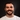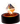Feb 01, 2019 · 4 min

# ReasonML vs TypeScript: comparing their type systems

#comparison
#reasonml
#typescript
#javascriptSeif Ghezala@seifghezala

A type is a labeled set of constraints that can be imposed on a value. A type system analyses values in a piece of code, and validates them against their types. JavaScript has a type system, but it’s dynamic. This is one of the key advantages of using the language, providing an incredible productivity gain.

Yet, we recently started to find more and more use-cases for statically typed languages that compile to JavaScript. TypeScript and ReasonML are two leaders of this trend that started a couple of years ago. They both compile to JavaScript but have different type systems. TypeScript was built as a superset of JavaScript. While Reason was built as an extension to the functional programming language OCaml.

In this article, we will put these two type systems under a microscope, studying some of their differences and similarities. It’s in no way intended to be a dance-off between Reason and TypeScript. Rather it’s a basic and objective comparison of their type systems (in case you were planning to call me out on this 😛). It’s also not a comprehensive analysis. There are other interesting points of comparison that this article doesn’t treat.

To run the code-snippets in the article, I suggest to use the following tools:

• JavaScript: the browser console.

• Reason: sketch.sh.

• In Reason, all variables are declared with the let keyword. In TypeScript, just like in JavaScript, you can use var , let or const . Since this is not the focus of the article, I will be merely using let in all the examples.

### Type checking time

You can classify type systems into 3 categories, based on their time of type validation:

#### Dynamic typing: JavaScript

Dynamically-typed languages don’t check for the types until run-time. Let’s look at the following example in JavaScript:

``let name = "John";``

At compile-time, only the declaration of the variable name is considered. At that point, the variable does not have a type associated with it.

At run-time, we bind the value “John” with the variable name and the variable is then considered to be a string.

#### Static typing: Reason

Statically-typed languages check for types every time the program is compiled. You get feedback about the type correctness even before running it.

Let’s try the same code snippet in Reason:

``let name = "John";``

In Reason, the variable name is associated with the type string before running the code.

You might ask: We didn’t specify any type here, so how can Reason determine that name is of type string?

This is called type inference. Languages such as Reason can infer the type of almost every value without you having to specify it:

``let sum = (a, b) => a + b;``

If you run this example on sketch.sh, you will see that Reason was able to determine that a , b and the return of sum are all integers.

Reason managed to do that because we used + , which is an operator specific to integers. In fact, each type has its own specific operators.

Gradual typing lies somewhere in-between the two previous type systems:

``let name = "John";``

If you hover over the variable name in the playground, you’ll see that TypeScript managed to associate name with the string variable.

In fact, TypeScript provides type inference as well:

``let sum = (a, b) => a + b;``

If you hover over sum in the playground, you will see something like this:

``let sum: (a: any, b: any) => any;``

In this case, TypeScript didn’t associate a particular type to either a , b or the return value of sum . The types of the sum function’s arguments or return could be anything. These types will be determined in runtime when executing the function. This is due to the + operator, which is not specific to any particular type in TypeScript or JavaScript.

This gradual typing is exactly what distinguishes TypeScript from Reason. In a gradually-typed language such as TypeScript, some declarations will have their types checked during compile-time and others will have their types checked at run-time.

### Type annotation

In the previous section, we relied on the type system’s inference ability. However, both TypeScript and Reason support explicit typing through type annotations.

They have exactly the same syntax:

``VARIABLE_NAME: TYPE;``

Here’s an example that works in both TypeScript and Reason:

``let name: string = "John";``

For functions, you can annotate both the parameters and the return value.

Let’s look again at the sum example.

In TypeScript:

``let sum = (a: number, b: number): number => a + b;``

In Reason:

``let sum = (a:int, b:int):int => a + b;``

### Common basic types

Regardless of how types are checked, TypeScript and Reason share the following basic types:

• Boolean: (bool in Reason, boolean in TypeScript)

• String

### Numbers

Just like in JavaScript, TypeScript has a single type for numbers:

``````let a = 1; // type: number
let b = 1.0; // type: number``````

Reason distinguishes between Integers and Floats:

``````let a = 1;    // type: integer
let b = 1.0;  // type: float``````

Reason has different operators for these types. Reason won’t even let you perform operations between a and b unless you convert one of them to the type of the other.

``````a + b;
^
Error: this expression has type float but an expression was expected of type int.``````

### Strings and characters

Both TypeScript and Reason share the string type. However, Reason has an extra type for single characters: `char`.

To distinguish between them, a double-quoted text is considered to be of type string and single-quoted text is considered to be a character.

``````let someString = "hello"; // type: string

let someChar = 'c'; // type: character``````

### Non-existing values

#### void & unit

Let’s look at functions that don’t return anything. How would you represent that in TypeScript or Reason?

In TypeScript, the return type of the function is void:

``````let greetName = (name: string): void => {
console.log(`Hello \${name}`);
};``````

The equivalent of void in Reason is unit:

``````let greetName = (name:string):unit => {
print_endline(“Hello” ++ name);
}``````

#### null, undefined & option

Let’s implement a function that finds the first string item in an array that satisfies a given condition:

``````let find = (arr: string[], condition: (item:string):boolean):string => {
return arr.find(condition);
}``````

Let’s play around with it:

``````let array = ["foo", "bar"];
// (1)
find(array, (item) => item.length === 3); // output: "foo"
// (2)
find(array, (item) => item.length === 1); // output: undefined``````

In the first example, a string item is returned just like we promised in our function annotation.

In the second example, an item is not found and undefined is returned instead. This is still correct in TypeScript since undefined is a subtype of all the other types. In other words, you can assign undefined to a number or a string variable. The same applies to null. In these use cases, TypeScript handles non-existing values with either the undefined or null type.

Reason approaches this kind of non-existing values differently. Our find function will have an option(string) return type.

Here’s how we would implement the same function in Reason:

Note: For simplicity, the function receives a list here instead of an array. A list is pretty much an immutable version of an array.

``````let find = (l: list(string), condition): option(string) => {
switch (List.find(condition, l)) {
| found => Some(found)
| exception Not_found => None
};
};``````

The returned value can then be handled through pattern matching to check whether a value is returned:

``````let list = ["foo", "bar"];
let condition = item => String.length(item) === 3;
switch (find(list, condition)) {
| Some(value) => print_endline(value)
};``````

### Tuples

A tuple represents a couple of values with specific types.

Here’s how we would define and use a type in TypeScript:

``let person: [string, number] = ["Marie", 24];``

Tuples exist in Reason as well and have a slightly different syntax:

``let person:(string, int) = ("Marie", 24);``

### Records vs Interfaces

In real-world applications, we often deal with values that can’t be expressed with just the previously seen ones. Restricting values to a certain shape provides more confidence in dealing with these values.

In TypeScript, it’s possible to create shapes of values through Interfaces:

``````interface Car {
color: string;
year: number;
brand: string;
}

let printCar = (car: Car): void => {
console.log(car.color, car.year, car.brand);
};

printCar({ color: "black", year: 2017, brand: "Tesla" });``````

The same can be achieved in Reason through Records:

``````type car = {
color: string,
year: int,
brand: string,
};

let printCar = (c: car): unit => {
print_endline(c.color ++ string_of_int(c.year) ++ c.brand);
};

printCar({color: "black", year: 2017, brand: "Tesla"});``````

### Variants vs Enums

Values are sometimes restricted to a specific set.

In TypeScript, it’s possible to define such sets through enums:

``````enum Answer {
YES,
NO,
}

let printResponse = (r: Answer): void => {
console.log("Yes");
} else {
console.log("No");
}
};``````

The same can be achieved in Reason through Variants:

``````type answer =
| YES
| NO;